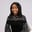Related Tags

python
blockchain

# How to create a simple blockchain with PythonAbiola Esther

### Introduction

Blockchain brought about an innovative way of solving problems using decentralized technology. It involves a series of cryptographic blocks linked together through hashes. The following are some characteristics of blockchain:

• Immutability: Data stored on a blockchain cannot be changed.
• Decentralized technology: No single authority controls the system.
• Secure: All the records in the blockchain are individually encrypted.

We'll mainly focus on implementing the basics of a blockchain with Python. First, we start with the Block class.

### Block class

We use the concept of object-oriented programming to create a class that would consist of the different properties of a block. Each block contains a cryptographic hash of the previous block, a timestamp, and transaction data.

class Block:

def __init__(self,  data, previous_hash):
self.timestamp =  datetime.now()
self.data = data
self.hash = self.calc_hash()
self.previous_hash = previous_hash
self.next = None
Blockchain.py
• timestamp: We use the datetime module to create get the current date and time and create the block.
from datetime import datetime
Blockchain.py

From datetime.now(), we get the timestamp property.

• data: We'll store the data in a string format.
• hash: For each block, we generate a new hash using the SHA-256 hashing algorithm.

We need to import the hashlib library for encryption.

import hashlib
def calc_hash(self):
hash_str = "We are going to encode this string of data!".encode('utf-8')
return hashlib.sha256(hash_str).hexdigest()
Blockchain.py

The function accepts the data as a string and then encodes it, we use the SHA256() function for encryption after which it is converted into a hexadecimal string.

• previous_hash: This contains the hash value of the previous block.

### Blockchain class

A blockchain consists of different blocks that are linked together. To link each block together, we use a linked list.

We initialize the list with the head node and next pointer.

class Blockchain:
def __init__(self):
self.next = None
Blockchain.py

Next, we create add_block to add each successive block to the chain.

 def add_block(self, data):

else:

# starts a loop
while current.next:
current = current.next

# stores the previous has for the next block
previous_hash = current.hash
current.next = Block(data, previous_hash)
Blockchain.py

For this, we create a loop that assigns the block data for the current node. print_blockchain prints the block data.

    def print_blockchain(self):

print("The blockchain is empty")
return

else:
while current:
print("Timestamp:", current.timestamp)
print("Data:", current.data)
print("Hash:", current.hash)
print("Previous hash:", current.previous_hash)
print("--------------->")

current = current.next
Blockchain.py
bitcoin = Blockchain()

bitcoin.print_blockchain()
Blockchain.py

In the above snippet, we create a new blockchain, then add some new blocks to it.

From this, we print out the blocks that we added.

import hashlib
from datetime import datetime
class Block:

def __init__(self,  data, previous_hash):
self.timestamp =  datetime.now()
self.data = data
self.previous_hash = previous_hash
self.hash = self.calc_hash()
self.next = None

def calc_hash(self):
hash_str = "We are going to encode this string of data!".encode('utf-8')
return hashlib.sha256(hash_str).hexdigest()

class Blockchain:

def __init__(self):
self.next = None

else:

# loop to the last node of the linkedlist
while current.next:
current = current.next

# stores the previous has for the next block
previous_hash = current.hash
current.next = Block(data, previous_hash)

def print_blockchain(self):

print("The blockchain is empty")
return

else:
while current:
print("Timestamp:", current.timestamp)
print("Data:", current.data)
print("Hash:", current.hash)
print("Previous hash:", current.previous_hash)
print("--------------->")

current = current.next

bitcoin = Blockchain()

bitcoin.print_blockchain()
Blockchain.py

RELATED TAGS

python
blockchain

CONTRIBUTORAbiola Esther
RELATED COURSES

View all Courses

Keep Exploring

Learn in-demand tech skills in half the time